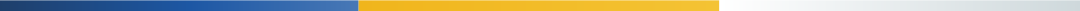# 离婚房产分割计算方法一览表（2021离婚新婚姻法）

2007年风和日丽的一天？？？？？

（本金180000元，利息45419元）a=首付款金额

b=按揭贷款金额

c=贷款利息

d=离婚时房产价值（可由双方协商确认，或由专业机构评估定价）

e=夫妻共同还贷本息金额（可在贷款银行中查询记录）

☀第二，计算夫妻共同还贷部分占房价款的比例y

y=e÷（a+b+c）

☀第三，计算找补金额za=首付款金额=122800元

b=按揭贷款金额=180000元

c=贷款利息=45419元

d=离婚时房产价值=580000元

e=夫妻共同还贷本息金额=203360.55元

y=e÷（a+b+c）=203360.55÷（122800+180000+45419）=58.4%

z=d×y÷2=580000×58.4%÷2=169360元；

z=d-d×y÷2

=580000-580000×58.4%÷2=410640元

《中华人民共和国婚姻法》

（一）工资、奖金；

（二）生产、经营的收益；

（三）知识产权的收益；

（四）继承或赠与所得的财产，但本法第十八条第三项规定的除外；

（五）其他应当归共同所有的财产。